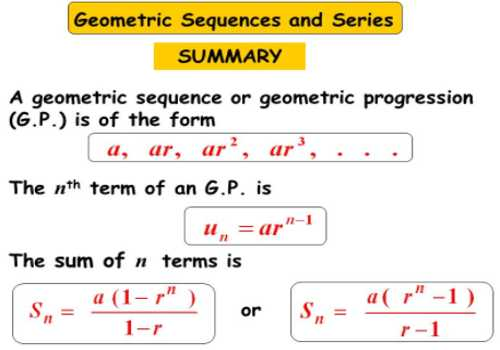A sequence of numbers is said to be Geometric Progression if the ratio of any term and its preceding term is always a constant quantity. It is a sequence of non-zero terms in which each succeeding term is obtained by multiplying its preceding term by a constant.

Definition of Geometric Progression:

A sequence of non-zero number is said to be in Geometric Progression (abbreviated as G.P.) if each term, after the first, is obtained by multiplying the preceding term by a constant quantity (positive or negative).

In mathematics, a geometric progression (sequence) (also inaccurately known as a geometric series) is a sequence of numbers such that the quotient of any two successive members of the sequence is a constant called the common ratio of the sequence.

The geometric progression can be written as:

ar0 = a, ar1 =ar, ar2, ar3, …

where r ≠ 0, r is the common ratio and a is a scale factor(also the first term).

The constant ratio is said to be the common ratio of the Geometric Progression and is denoted by dividing any term by that which immediately precedes it.

In other words, the sequence {a1, a2, a3, a4, ……………….., an, …………….. } is said to be in Geometric Progression, if an+1 / a = constant for all n ϵ N i.e., for all integral values of a, the ratio (an+1 / a) is constant.Examples

A geometric progression with common ratio 2 and scale factor 1 is

1, 2, 4, 8, 16, 32…

A geometric sequence with common ratio 3 and scale factor 4 is

4, 12, 36, 108, 324…

A geometric progression with common ratio -1 and scale factor 5 is

5, -5, 5, -5, 5, -5,…

The common ratio of a geometric sequence may be negative, resulting in an alternating sequence, with numbers switching from positive to negative and back. For instance:

(1, −3, 9, −27, 81, −243, …); is a geometric sequence with common ratio −3.

The behavior of a geometric sequence depends on the value of the common ratio.

If the common ratio is:

• Positive, the terms will all be the same sign as the initial term.
• Negative, the terms will alternate between positive and negative.
• Greater than 1, there will be exponential growth towards positive or negative infinity (depending on the sign of the initial term).
• 1, the progression is a constant sequence.
• Between −1 and 1 but not zero, there will be exponential decay towards zero.
• −1, the progression is an alternating sequence
• Less than −1, for the absolute values there is exponential growth towards (unsigned) infinity, due to the alternating sign.

Information Source: